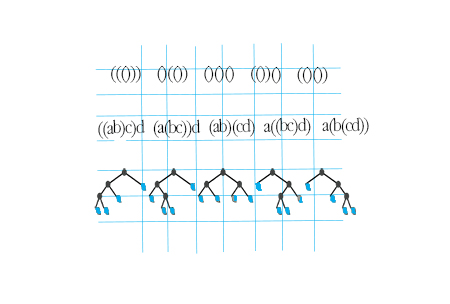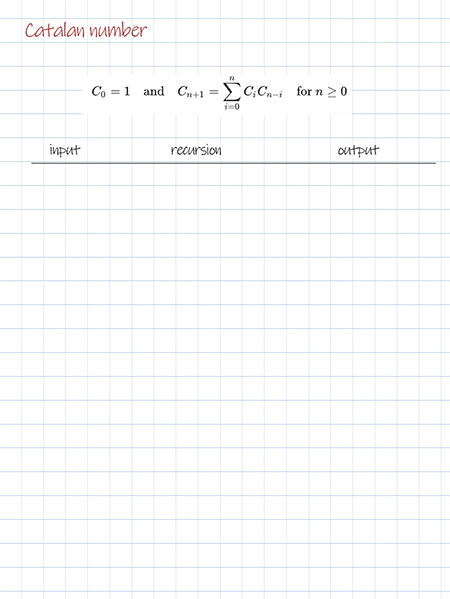Catalan numbers are a sequence of natural numbers that follow the formula showing below.

The first few Catalan numbers for n = 0, 1, 2, 3, … are 1, 1, 2, 5, 14, 42, 132, 429, 1430, 4862, …

Catalan numbers occur in many counting problems in combinatorics, such as count the number of ways to generate n pairs of valid parentheses, count the number of full binary trees with n+1 leaves. Given a number n , you can find n-th Catalan number using the following 3 implementations.### Iteration

This solution is applying the following alternative expression of Cn. It can be implemented with iteration. It has the best time complexity O(n).

## Python

### Dynamic programming

This solution uses the same expression as recursion. But it overcomes the overlapping in recursion by using dynamic programming technique tabulation. The result from previous calls are saved to a 2d array. They can be re-used for the following steps without calling the recursion. It improves the time complexity from exponential to O(n^2).

## Python

### Recursion

This solution is using the another alternative expression of Cn. It can be implemented with recursion. It has the worst time complexity O(2^n) with repeated calls.

## Doodle